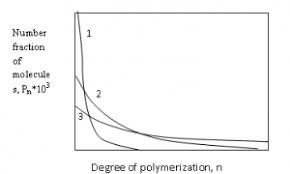## How to Calculate and Solve for Degree of Polymerization | PolymerThe image of degree of polymerization is represented above.

To compute for degree of polymerization, two essential parameters are needed and these parameters are Mean Number Molecular Weight (M’nand Unit Molecular Weight (m).

The formula for calculating degree of polymerization:

D.P. = M’n/m

Where:

D.P. = Degree of Polymerization
M’n = Mean Number Molecular Weight
m = Unit Molecular Weight

Let’s solve an example;
Find the degree of polymerization when the mean number molecular weight is 28 and the unit molecular weight is 4.

This implies that;

M’n = Mean Number Molecular Weight = 28
m = Unit Molecular Weight = 4

D.P. = 28/4
D.P. = 7

Therefore, the degree of polymerization is 7.

Calculating the Mean Number Molecular Weight when the Degree of Polymerization and the Unit Molecular Weight is Given.

M’n = D.P (m)

Where;

M’n = Mean Number Molecular Weight
D.P. = Degree of Polymerization
m = Unit Molecular Weight

Let’s solve an example;
Find the mean number molecular weight when the degree of polymerization is 6 and the unit molecular weight is 4.

This implies that;

D.P. = Degree of Polymerization = 6
m = Unit Molecular Weight = 4

M’n = D.P (m)
M’n = 6 (4)
M’n = 24

Therefore, the degree of polymerization is 24.